In modular arithmetic, a quadratic residue for the modulus N is a number which can be expressed as the residue of a2 modulo N for some integer a. A quadratic non-residue of N is a number which is not a quadratic residue of N.

## Legendre symbol

When the modulus is a prime p, the Legendre symbol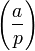$\left(\frac{a}{p}\right)$ expresses the quadratic nature of a modulo p. We write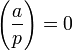$\left(\frac{a}{p}\right) = 0 \,$ if p divides a;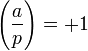$\left(\frac{a}{p}\right) = +1 \,$ if a is a quadratic residue of p;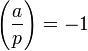$\left(\frac{a}{p}\right) = -1 \,$ if a is a quadratic non-residue of p.

The Legendre symbol is multiplicative, that is,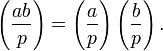$\left(\frac{ab}{p}\right) = \left(\frac{a}{p}\right)\left(\frac{b}{p}\right) . \,$

## Jacobi symbol

For an odd positive n, the Jacobi symbol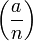$\left(\frac{a}{n}\right)$ is defined as a product of Legendre symbols$\left(\frac{a}{n}\right) = \prod_{i=1}^r \left(\frac{a}{p_i}\right)^{e_i} \,$

where the prime factorisation of n is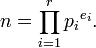$n = \prod_{i=1}^r {p_i}^{e_i} . \,$

The Jacobi symbol is bimultiplicative, that is,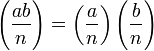$\left(\frac{ab}{n}\right) = \left(\frac{a}{n}\right)\left(\frac{b}{n}\right) \,$

and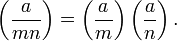$\left(\frac{a}{mn}\right) = \left(\frac{a}{m}\right)\left(\frac{a}{n}\right) . \,$

If a is a quadratic residue of n then the Jacobi symbol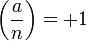$\left(\frac{a}{n}\right) = +1$, but the converse does not hold. For example,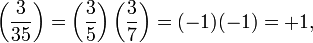$\left(\frac{3}{35}\right) = \left(\frac{3}{5}\right)\left(\frac{3}{7}\right) = (-1)(-1) = +1 , \,$

but since the Legendre symbol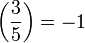$\left(\frac{3}{5}\right) = -1$, it follows that 3 is a quadratic non-residue of 5 and hence of 35.